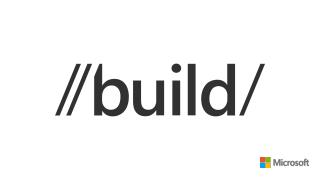DownloadDownload PresentationDeveloping Neural Networks using Visual Studio

# Developing Neural Networks using Visual Studio

Download Presentation## Developing Neural Networks using Visual Studio

- - - - - - - - - - - - - - - - - - - - - - - - - - - E N D - - - - - - - - - - - - - - - - - - - - - - - - - - -
##### Presentation Transcript

1. Developing Neural Networks using Visual Studio James McCaffrey Microsoft Research 2-401

2. Agenda • Slide 1: What types of problems does a neural network solve? • Slide 2: What exactly is a neural network? • Slide 3: How does a neural network actually work? • Slide 4: Understanding activation functions. • Slide 5: Alternatives to neural networks. • Slide 6: Understanding neural network training. • Slide 7: Neural network over-fitting. • Slide 8: Developing with Visual Studio. • Slide 9: Summary and resources.

3. What type of problems does a neural network solve? Training data Independent variables/predictors/attributes/regressors/x-values “The thing to classify (predict)”/ dependent variable/y

4. What is a neural network? Age 38 3.8 input hidden output Income 51,000 5.1 0.43 Politics Sex M -1.0 0.20 Dem 0.0 0.37 Religion Pres 1.0 0.0

5. The perceptron building block 0.10 W0 = 4.0 b = 2.0 a. (0.1)(4.0) + (0.2)(-5.0) + (0.3)(6.0) = 1.2 b. 1.2 + 2.0 = 3.2 c. Activation(3.2) = 0.73 d. ?? = 0.73 0.20 ?? W1 = -5.0 Technical note: most neural network literature treats the bias as a weight that has a dummy input which is always a 1.0 value. 0.30 W2 = 6.0

6. Four most common activation functions • Logistic sigmoid • Output between [0, 1] • y = 1.0 / (1.0 + e–x) • Hyperbolic tangent • Output between [-1, +1] • y = tanh(x) = (ex – e-x) / (ex + e-x) • Heaviside step • Output either 0 or 1 • if (x < 0) then y = 0 else if (x >= 0) then y = 1 • Softmax • Outputs between [0, 1] and sum to 1.0 • y = (e-xi) / Σ (e-xj)

7. Alternatives to neural networks • The six main alternatives to using a neural network • 1. Linear regression: assumes data can be modeled as y = ax1 + bx2 + . . + k • 2. Logistic regression: assumes data can be modeled as y = 1.0 / ( 1.0 + e-(ax1 + bx2 + . . + k) ) • 3. Naive Bayes: assumes input data are all independent, output is binary. • 4. Decision trees: do not work well for complex data, assumes binary output. • 5. Adaptive boosting: relatively new and effectiveness not well understood, assumes binary output. • 6. Support vector machines: extremely complex implementation, assumes binary output.

8. Alternatives to neural networks • Neural networks pros and cons • Pro: can model any underlying math equation! • Pro: can handle multinomial output without resorting to tricks. • Con: moderate complexity, requires lots of training data. • Con: must pick number hidden nodes, activation functions, input/output encoding, error definition. • Con: must pick training method, training “free parameters,” (and over-fitting defense strategy).

9. Training • Back-propagation • Fastest technique. • Does not work with Heaviside activation. • Requires “learning rate” and “momentum.” • Genetic algorithm • Slowest technique. • Generally most effective. • Requires “population size,” “mutation rate,” “max generations,” “selection probability.” • Particle swarm optimization • Good compromise. • Requires “number particles,” “max iterations,” “cognitive weight,” “social weight.”

10. Avoiding model over-fitting • What is it? • Symptom: Model is great on predicting existing data, but fails miserably on new data. • Roulette example: red, red, black, red, red, black, red, red, black, red, red, ?? • A serious problem for all classification/prediction techniques, not just neural networks. • Five most common techniques • Use lots of training data. • Train-Validate-Test (early stop when error on validate set begins to increase). • K-fold cross validation. • Repeated sub-sampling validation. • Jittering: deliberately adding noise data to make over-fitting impossible. • Quite a few exotic techniques also available (weight penalties, Bayesian learning, etc.).

11. Weka

12. TLC

13. TLC API

14. Visual Studio

15. Demo: the famous Iris problem

16. Summary • Existing neural network tools are difficult or impossible to integrate into a software system. • Commercial and Open Source API libraries work well for some machine learning tasks but are extremely limited for neural networks. • To develop neural networks using Visual Studio you must understand seven core concepts: feed-forward, activation, data encoding, error, training, free parameters, and over-fitting. • Once the concepts are mastered, implementation with Visual Studio is not difficult (but not easy either).

17. Resources • Concepts: • ftp://ftp.sas.com/pub/neural/FAQ.html#questions • Weka: • http://www.cs.waikato.ac.nz/ml/weka/ • Custom C#: • http://msdn.microsoft.com/en-us/magazine/jj190808.aspx Special enhanced demo code for Build 2013 attendees: http://www.quaetrix.com/Build2013.html

18. Thank You! Session 2-401 • Developing neural networks using Visual Studio. • 2013 Build Conference • June 25–28, 2013 • San Francisco, CA • Dr. James McCaffrey • Microsoft Research • jammc@microsoft.com

19. Acer Iconia W3, Surface Pro, and Surface Type Cover Get your goodies Device distribution starts after sessions conclude today (approximately 6:00pm) in the Big Room, Hall D. If you choose not to pick up your devices tonight, distribution will continue for the duration of the conference at Registration in the North Lobby.

20. Required Slide *delete this box when your slide is finalized Your MS Tag will be inserted here during the final scrub. Evaluate this session • Scan this QR codeto evaluate this session and be automatically entered in a drawing to win a prize!